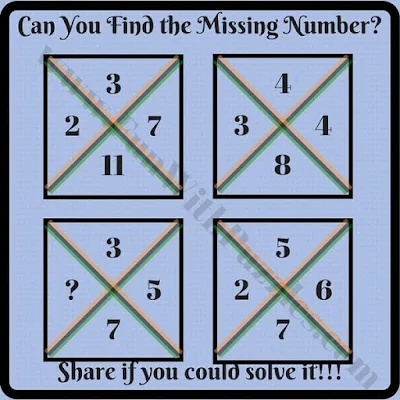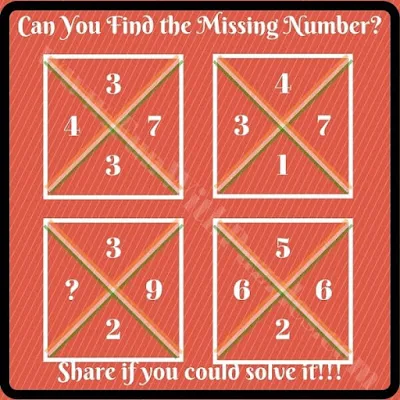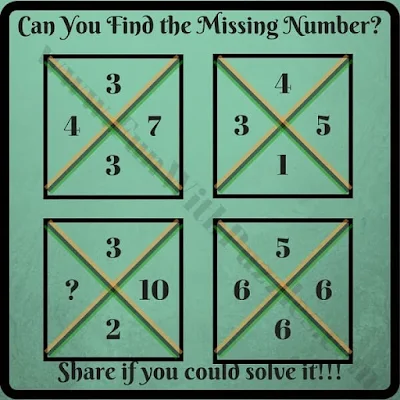Today's Cool Math Brain Teasers is an extension of earlier posted similar Cross Square Math Brain Teasers. We have been posting Math Brain Teasers with numbers around different mathematical shapes. One should definitely check these Math Brain Teasers after solving today's Cool Math Brain Teasers.
In these Cool Maths Brain Teasers, there are four numbers given in each of the squares which are divided by a cross. These four numbers relate to each other with mathematical operations. One has to find this relationship and then find the missing number in one of the squares.
So are you ready to find the missing number which will replace the question mark? Answers to these puzzles are given at the end. Do look out for the answers only to verify your solutions. Also, post your answers in the comment section along with your explanations to solve these puzzles.2. Can you find the missing number in this math puzzle?4. Can you calculate the value of the missing number?5. Can you calculate the value of the missing number?

Also, check out the following Maths and Logical Brain Teasers to tickle your brain

## List of Maths and Logical Brain Teasers

1. 5 Awesome Brainteasers To Test Your Mental Sharpness: These are the puzzles in which you have to find the parking number in the given picture. These puzzles will test your Maths as well as Logical skills.

2. Dead Wife Murder Crime Detective Mystery Puzzle: It's time to play a detective and solve the murder mystery. Use your Logical Reasoning skills to solve this Mystery Puzzle.

3. Easy Mathematical Puzzle Questions with Answers for Students: If you found the earlier mentioned puzzle tough, then try out these easy Maths Puzzles.

4. Cool Maths Quiz for Kids with Answers: This contains another set of cool Mathematical Puzzles which are very easy to solve.

## Answers of Cool Maths Brain Teasers

To understand the answers to these cool maths brain teasers, let's first decide on the notations.

Let's say A, B, C, and D to the numbers starting from the top number and then going clockwise.

A1. 7
The logical Reasoning used in this brainteaser is as below
D x A = CB in other words
D x A = 10C + B

A2. 2
The logical Reasoning used in this brainteaser is as below
A+C = BxD

A3. 15
The logical Reasoning used in this brainteaser is as below
A+D = B+C

A4. 1
The logical Reasoning used in this brainteaser is as below
D+B - (A+C) = 5

A5. 0
The logical Reasoning used in this brainteaser is as below
A + B + D - C = 11